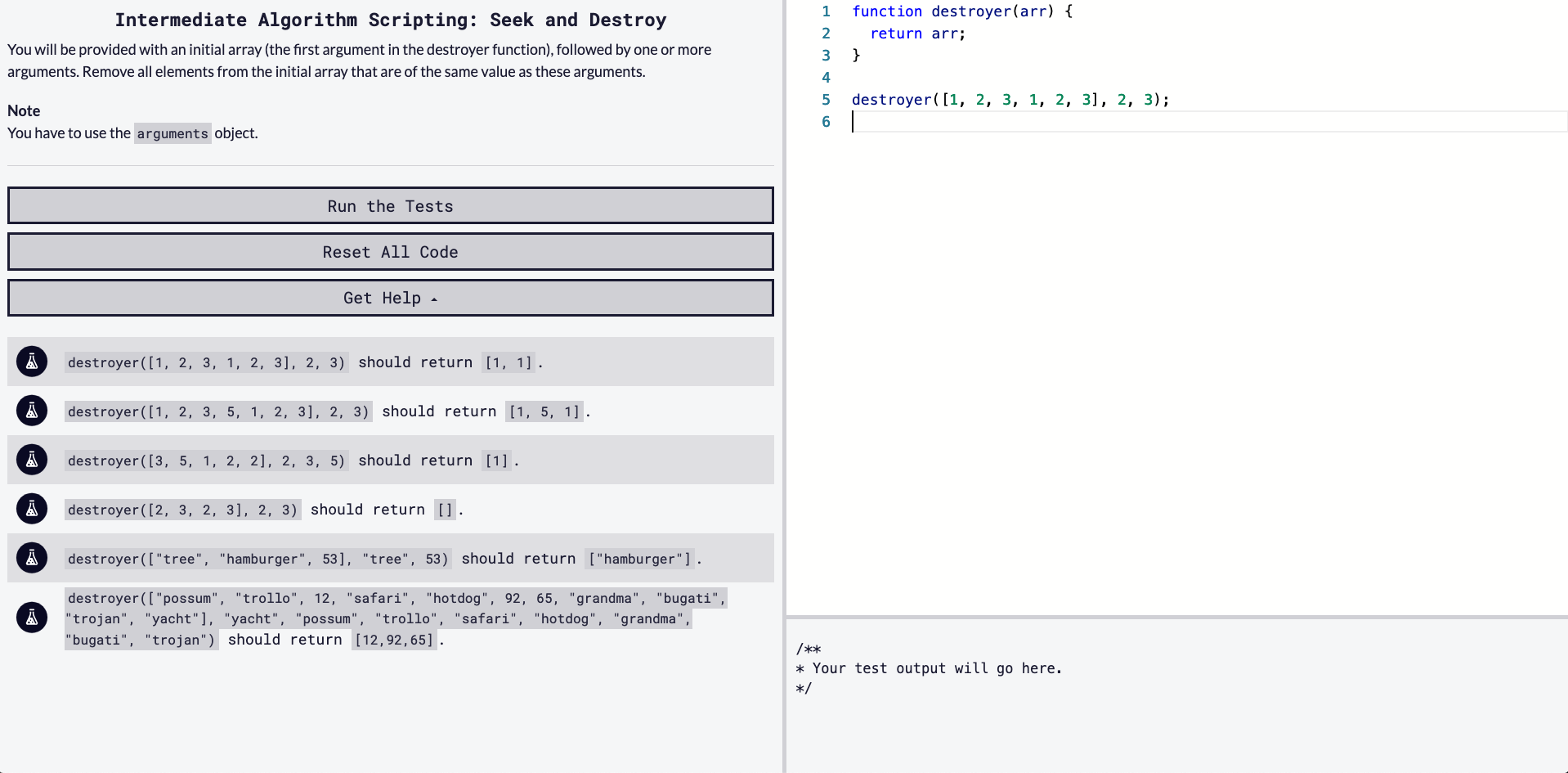## DEV Community

Viren B

Posted on • Originally published at virenb.cc

# Solving "Seek and Destroy" / freeCodeCamp Algorithm ChallengesLet's solve freeCodeCamp's intermediate algorithm scripting challenge, 'Seek and Destroy'.

### Starter Code

``````function destroyer(arr) {
return arr;
}

destroyer([1, 2, 3, 1, 2, 3], 2, 3);
``````

### Instructions

You will be provided with an initial array (the first argument in the destroyer function), followed by one or more arguments. Remove all elements from the initial array that are of the same value as these arguments.

Note

You have to use the `arguments` object.

### Tests

``````destroyer([1, 2, 3, 1, 2, 3], 2, 3) should return [1, 1].
destroyer([1, 2, 3, 5, 1, 2, 3], 2, 3) should return [1, 5, 1].
destroyer([3, 5, 1, 2, 2], 2, 3, 5) should return .
destroyer([2, 3, 2, 3], 2, 3) should return [].
destroyer(["tree", "hamburger", 53], "tree", 53) should return ["hamburger"].
destroyer(["possum", "trollo", 12, "safari", "hotdog", 92, 65, "grandma", "bugati", "trojan", "yacht"], "yacht", "possum", "trollo", "safari", "hotdog", "grandma", "bugati", "trojan") should return [12,92,65].
``````

# Our Approach

• The function takes two or more arguments. `arr` is an array and it can be followed by one or more arguments. Looking at the tests, these additional arguments are numbers or strings.
• We need to evaluate `arr` items against the other arguments to see if there are any matching values.
• We have to use the `arguments` object.
• We need to return an array

Now that we understand what we are given and what we want to output, let's see how we can solve this.

We can start by understanding the `arguments` object.

#### MDN: The arguments object

"arguments is an Array-like object accessible inside functions that contains the values of the arguments passed to that function."

For example,

``````function destroyer(arr) {
console.log(arguments);
}

destroyer([1, 2, 3, 1, 2, 3], 2, 3);
// [object Arguments] {
//  0: [1, 2, 3, 1, 2, 3],
//  1: 2,
//  2: 3
//}
``````

So, we have our arguments in an 'array-like' object.

I'm thinking we can convert `arguments` into an actual `Array` so we will have more built-in methods to potentially work with.

From the above mentioned MDN documentation, we learn how to convert `arguments` into an `Array`.

``````let args = Array.from(arguments);
// or
let args = [...arguments];
``````

We now have an array of all our arguments. The first item in our new array is an array where we want to check if the other items exist. I would like to separate the `arr` from the other arguments, so we can call a frequently used method, `slice()`.

#### MDN: Array.slice()

We can call it like the below to result in having the other arguments in their own array.

`const argsArray = [...arguments].slice(1);`

`destroyer([1, 2, 3, 1, 2, 3], 2, 3);`

This would give us `argsArray = [2, 3]`;

We can now prepare a for loop on the amount of `arr`'s length. We will have to check if each index in `arr` exists in our `argsArray`. If it is false, we can push it into a new empty array, and we would return the new array.

To check if the value exists in the array, we have one more `Array` method which we can use: `indexOf()`.

#### MDN: Array.indexOf()

When we compare, we will check the equality against -1 to see if the item is in the array or not (example below).

``````const nums = [1, 2, 3, 4];
console.log(nums.indexOf(20));
// -1
``````

So, some pseudo-code:

``````function destroyer(arr) {
create new array from arguments object but slice to remove arr
create new empty array to house unique values
for loop run arr.length of times
if each index of arr is not in new args array

return unique array
}
``````

# Our Solution

⚠️
⚠️

``````function destroyer(arr) {
const argsArray = [...arguments].slice(1);
const uniqueArray = [];
for (let i = 0; i < arr.length; i++) {
if (argsArray.indexOf(arr[i]) == -1) {
uniqueArray.push(arr[i]);
}
}
return uniqueArray;
}
``````